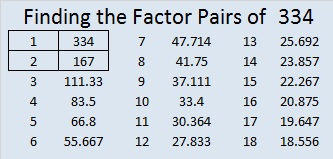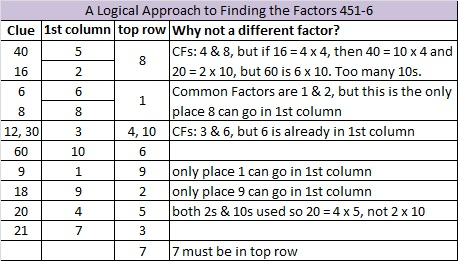# 334 and Level 6

• 334 is a composite number.
• Prime factorization: 334 = 2 x 167
• The exponents in the prime factorization are 1 and 1. Adding one to each and multiplying we get (1 + 1)(1 + 1) = 2 x 2 = 4. Therefore 334 has exactly 4 factors.
• Factors of 334: 1, 2, 167, 334
• Factor pairs: 334 = 1 x 334 or 2 x 167
• 334 has no square factors that allow its square root to be simplified. √334 ≈ 18.276Solving this Level 6 puzzle can be a little tricky: Is 1 or 2 the common factor for 6 and 8? Is 3 or 6 the common factor for 12 and 30? Is 4 or 8 the common factor for 40 and 16? In each case only one of those choices will make this puzzle work as a multiplication table? Can you figure out what those choices should be? Use logic to find the correct solution, not trial and error.Print the puzzles or type the factors on this excel file:  10 Factors 2014-12-22